# Multiobjective Optimization Multiobjective Optimization Some optimization problems have

• Slides: 29Multiobjective OptimizationMultiobjective Optimization • Some optimization problems have multiple objectives that required tradeoff decisions 2Pareto Optimality • At a Pareto optimal point, increasing one objective value decreases another • In multiobjective optimization, one point dominates another if 3Pareto Optimality 4Pareto Optimality: Pareto Frontier • A criterion space is a set of all outputs possible given a space of inputs • In a multidimensional optimization problem, the criterion space is m-dimensional. • In m-dimensional space, it is possible for there to be no clearly optimal point 5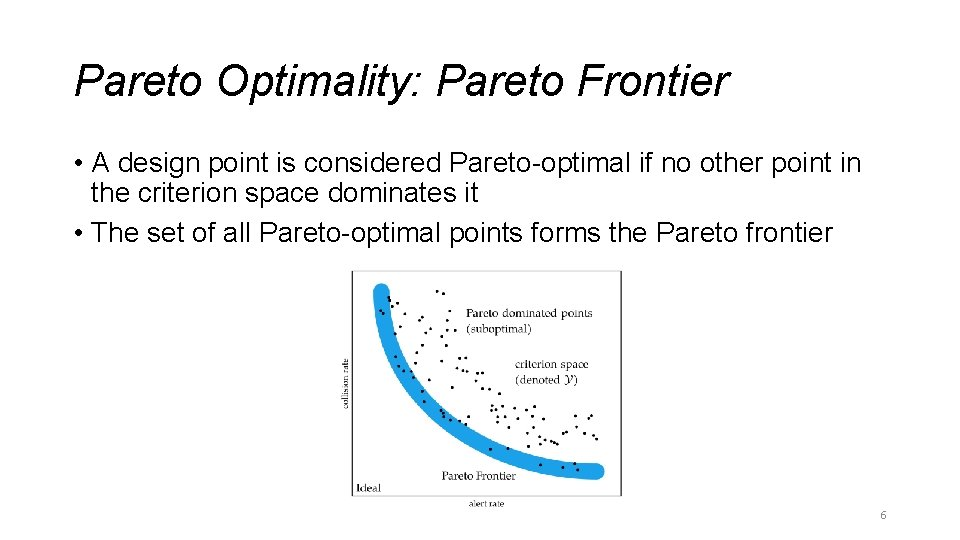Pareto Optimality: Pareto Frontier • A design point is considered Pareto-optimal if no other point in the criterion space dominates it • The set of all Pareto-optimal points forms the Pareto frontier 6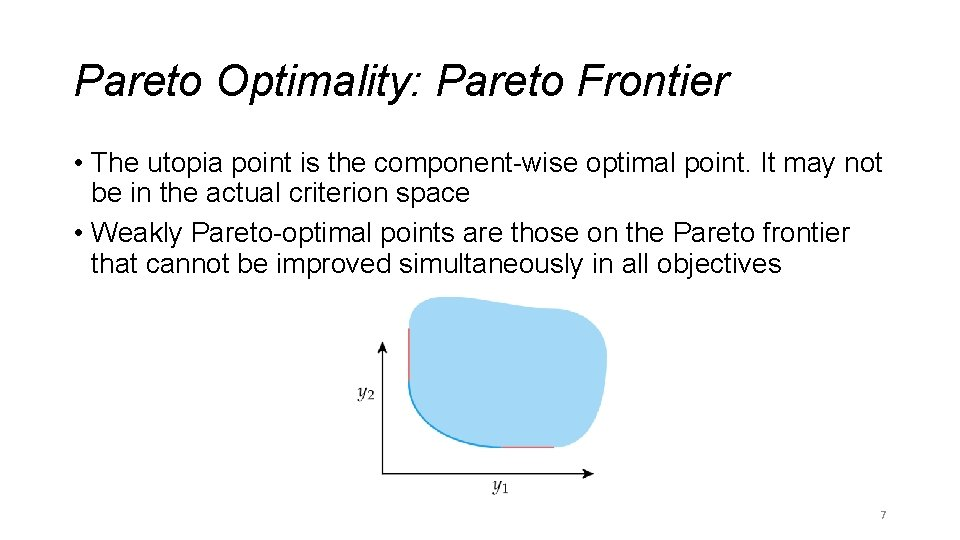Pareto Optimality: Pareto Frontier • The utopia point is the component-wise optimal point. It may not be in the actual criterion space • Weakly Pareto-optimal points are those on the Pareto frontier that cannot be improved simultaneously in all objectives 7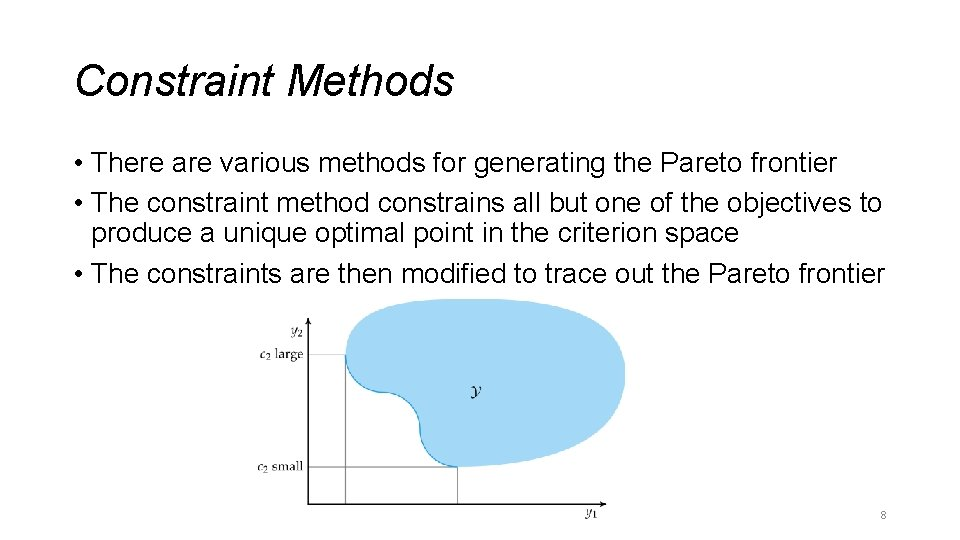Constraint Methods • There are various methods for generating the Pareto frontier • The constraint method constrains all but one of the objectives to produce a unique optimal point in the criterion space • The constraints are then modified to trace out the Pareto frontier 8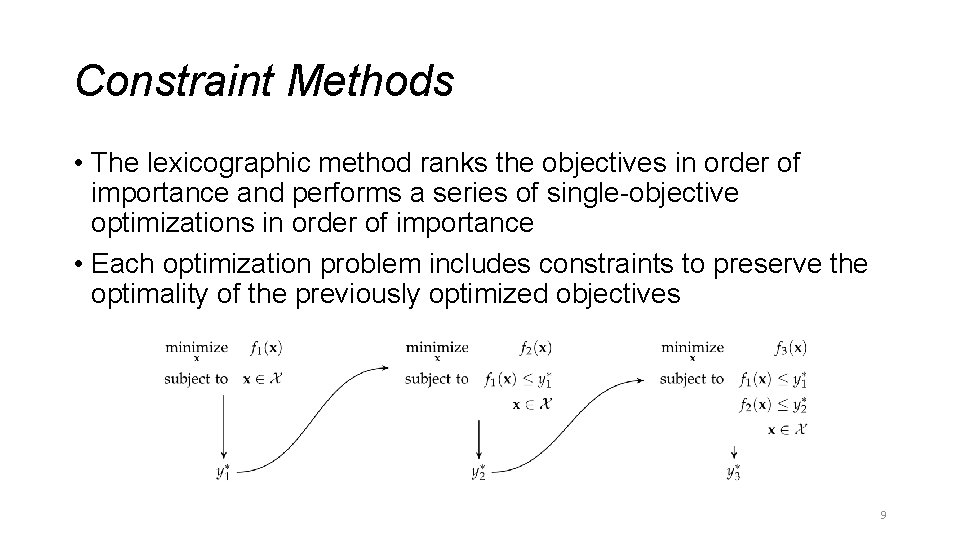Constraint Methods • The lexicographic method ranks the objectives in order of importance and performs a series of single-objective optimizations in order of importance • Each optimization problem includes constraints to preserve the optimality of the previously optimized objectives 9Weight Methods • A designer can encode preferences over the objectives as a vector of weights • The pareto frontier can be generated by sweeping over the space of possible weights • The weighted sum method combines multiple objective functions into one using a constant vector inner product 10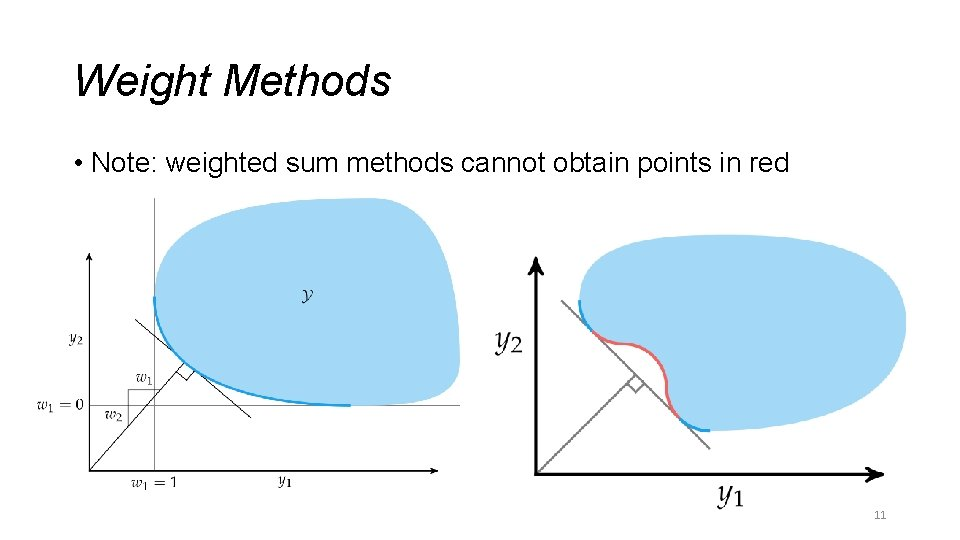Weight Methods • Note: weighted sum methods cannot obtain points in red 11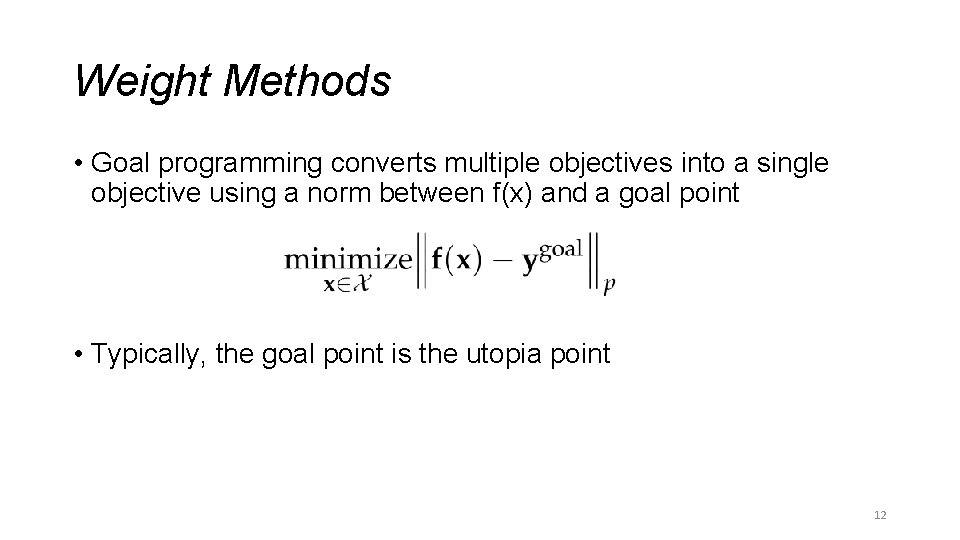Weight Methods • Goal programming converts multiple objectives into a single objective using a norm between f(x) and a goal point • Typically, the goal point is the utopia point 12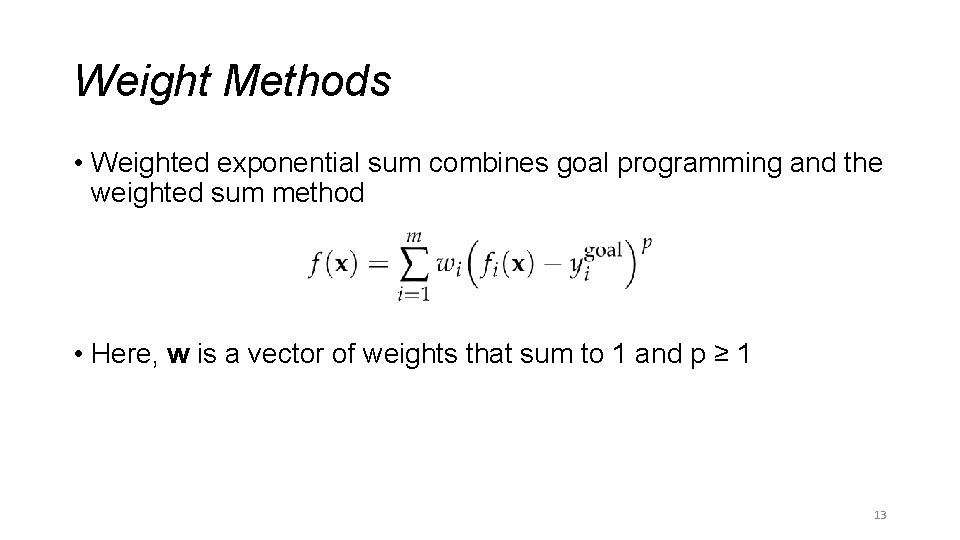Weight Methods • Weighted exponential sum combines goal programming and the weighted sum method • Here, w is a vector of weights that sum to 1 and p ≥ 1 13Weight Methods • Weighted exponential sum for different values of p 14Weight Methods • Previous methods cannot obtain points in nonconvex portions of the Pareto frontier • The exponential weighted criterion can provide Pareto-optimal points in nonconvex regions • Note: High values of p can lead to numerical overflow 15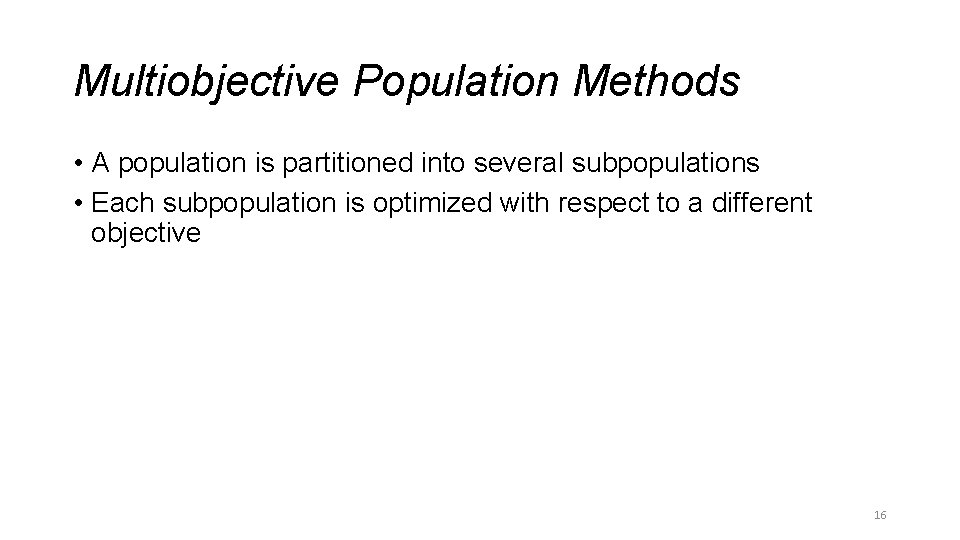Multiobjective Population Methods • A population is partitioned into several subpopulations • Each subpopulation is optimized with respect to a different objective 16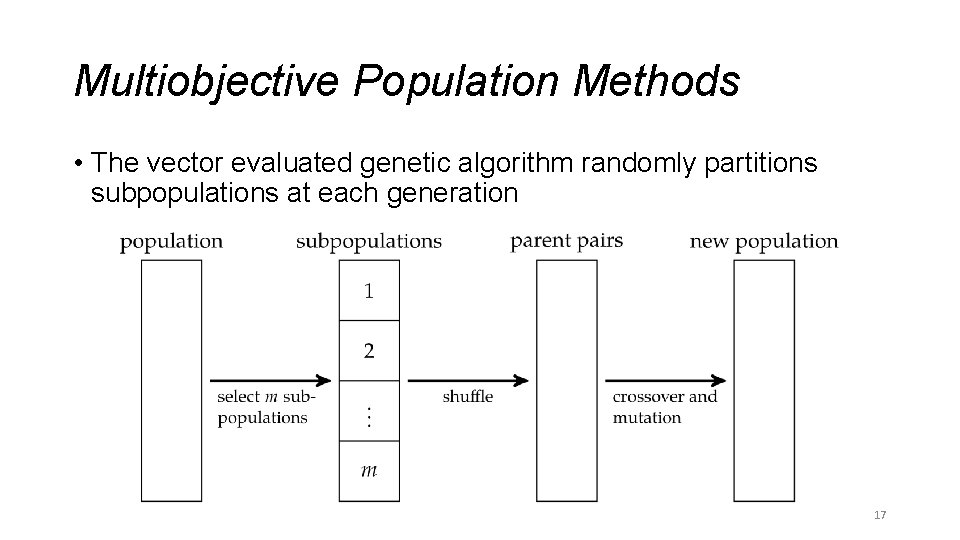Multiobjective Population Methods • The vector evaluated genetic algorithm randomly partitions subpopulations at each generation 17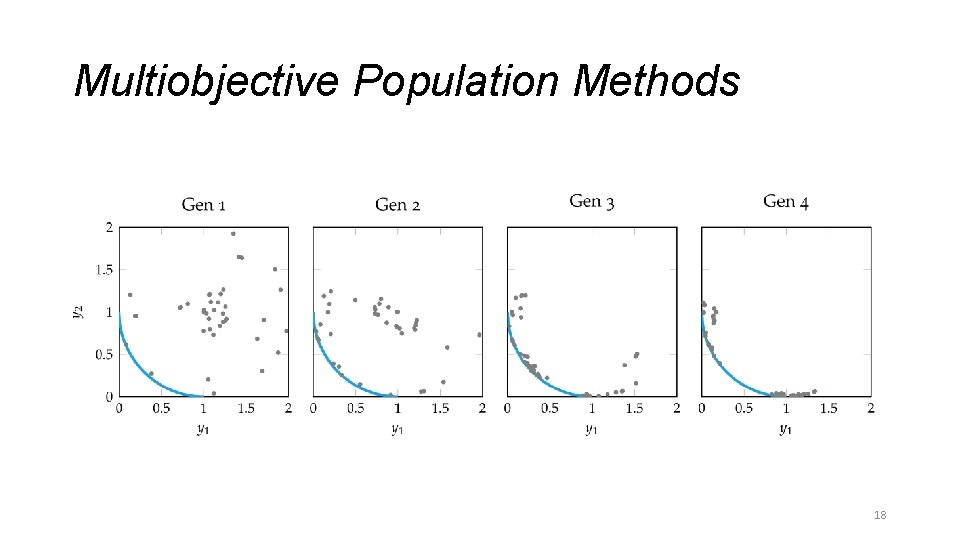Multiobjective Population Methods 18Multiobjective Population Methods • Nondomination ranking is used to rank individuals in the population 1. Nondominated individuals (Pareto-frontier) 2. Nondominated by any except those in (1) 3. Nondominated by and except those in (1) or (2) … 19Multiobjective Population Methods 20Multiobjective Population Methods • In multiobjective population methods, a population that approximates the Pareto frontier is called a Pareto filter • At every generation, dominant individuals are retained and dominated individuals are removed 21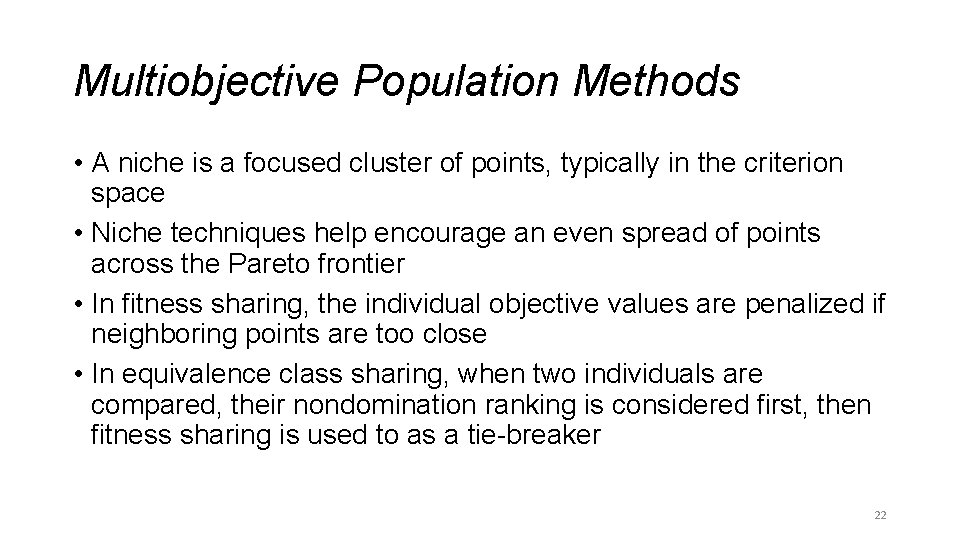Multiobjective Population Methods • A niche is a focused cluster of points, typically in the criterion space • Niche techniques help encourage an even spread of points across the Pareto frontier • In fitness sharing, the individual objective values are penalized if neighboring points are too close • In equivalence class sharing, when two individuals are compared, their nondomination ranking is considered first, then fitness sharing is used to as a tie-breaker 22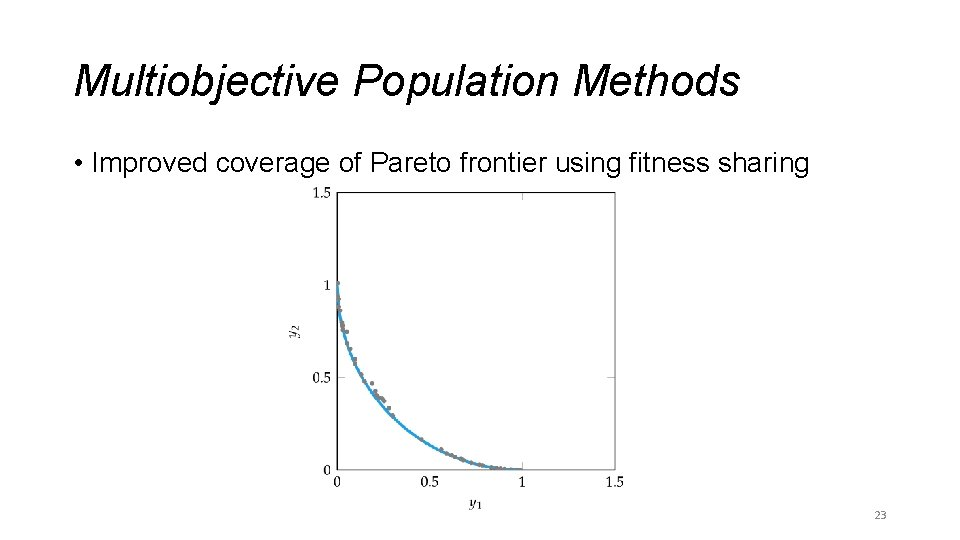Multiobjective Population Methods • Improved coverage of Pareto frontier using fitness sharing 23Preference Elicitation • If using a weighted sum method, how should the weights be determined? • Preference elicitation involves inferring a scalar-valued objective function from the preferences of experts about objective tradeoffs • A common approach is asking experts to choose preference between the optimized results of two weight distributions wa and wb. These distributions are continually adjusted until w aligns with user preferences 24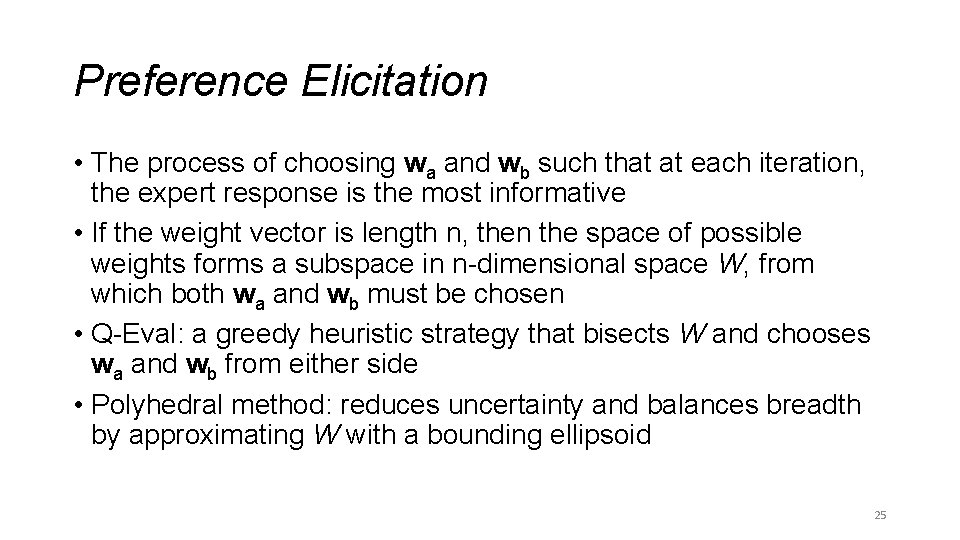Preference Elicitation • The process of choosing wa and wb such that at each iteration, the expert response is the most informative • If the weight vector is length n, then the space of possible weights forms a subspace in n-dimensional space W, from which both wa and wb must be chosen • Q-Eval: a greedy heuristic strategy that bisects W and chooses wa and wb from either side • Polyhedral method: reduces uncertainty and balances breadth by approximating W with a bounding ellipsoid 25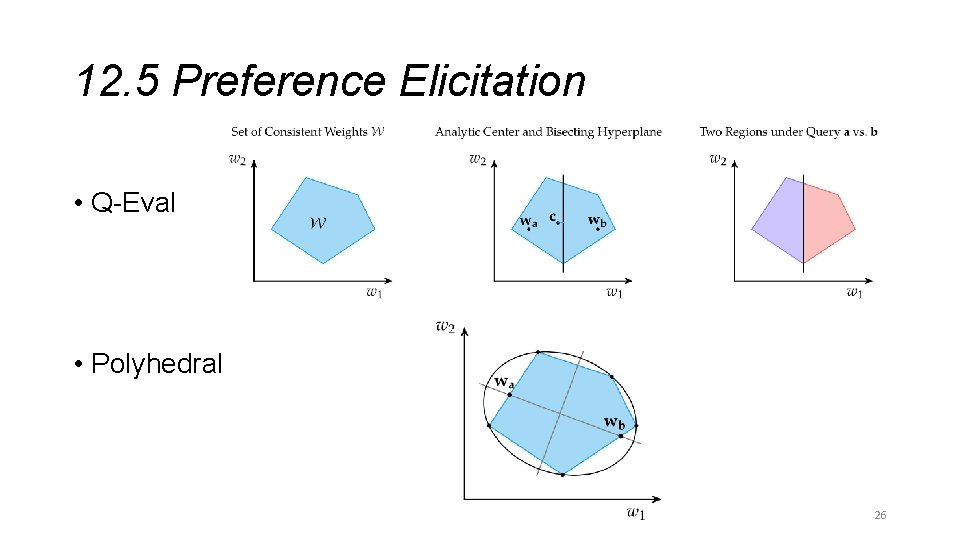12. 5 Preference Elicitation • Q-Eval • Polyhedral 26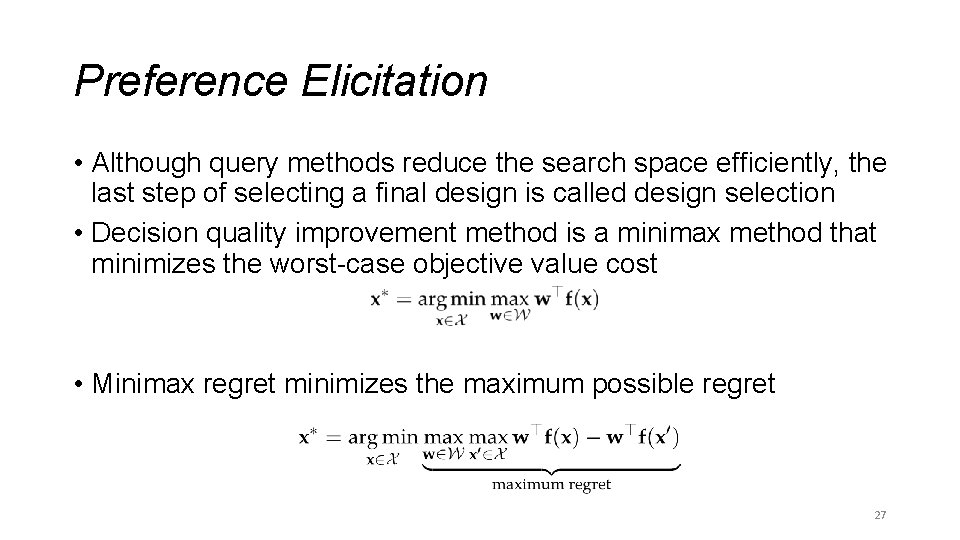Preference Elicitation • Although query methods reduce the search space efficiently, the last step of selecting a final design is called design selection • Decision quality improvement method is a minimax method that minimizes the worst-case objective value cost • Minimax regret minimizes the maximum possible regret 27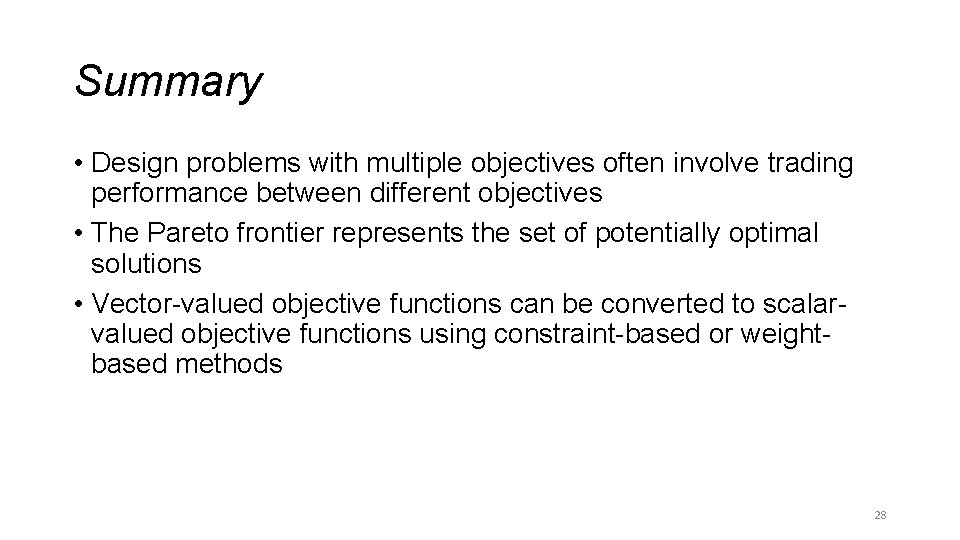Summary • Design problems with multiple objectives often involve trading performance between different objectives • The Pareto frontier represents the set of potentially optimal solutions • Vector-valued objective functions can be converted to scalarvalued objective functions using constraint-based or weightbased methods 28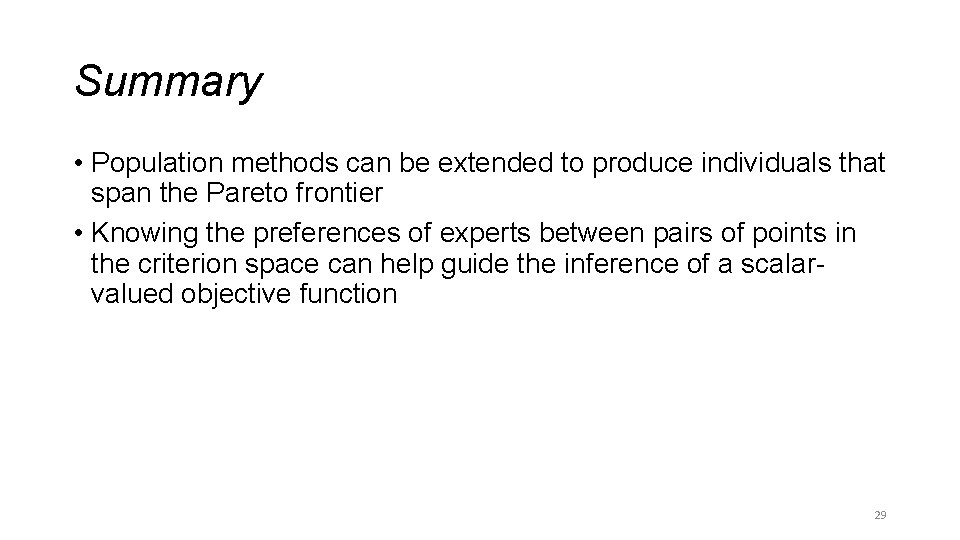Summary • Population methods can be extended to produce individuals that span the Pareto frontier • Knowing the preferences of experts between pairs of points in the criterion space can help guide the inference of a scalarvalued objective function 29